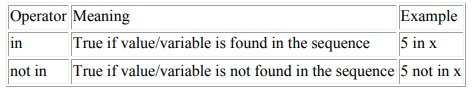Home | | Problem Solving and Python Programming | Type of operators in Python

# Type of operators in Python

Arithmetic operators, Comparison (Relational) operators, Logical (Boolean) operators, Bitwise operators, Assignment operators, Special operators.

Type of operators in Python

Arithmetic operators

Comparison (Relational) operators

Logical (Boolean) operators

Bitwise operators

Assignment operators

Special operators

### Arithmetic operators

Arithmetic operators are used to perform mathematical operations like addition,

subtraction, multiplication etc.

Arithmetic operators in PythonExample: Arithmetic operators in Python

x = 15

y = 4

# Output: x + y = 19

print('x + y =',x+y)

# Output: x - y = 11

print('x - y =',x-y)

# Output: x * y = 60

print('x * y =',x*y)

# Output: x / y = 3.75

print('x / y =',x/y)

# Output: x // y = 3

print('x // y =',x//y)

# Output: x ** y = 50625

print('x ** y =',x**y)

When you run the program, the output will be:

x + y = 19

x - y = 11

x * y = 60

x / y = 3.75

x // y = 3

x ** y = 50625

### Comparison operators

Comparison operators are used to compare values. It either returns True or False

according to the condition.Example: Comparison operators in Python

x = 10

y = 12

# Output: x > y is False

print('x > y is',x>y)

# Output: x < y is True

print('x < y is',x<y)

# Output: x == y is False

print('x == y is',x==y)

# Output: x != y is True

print('x != y is',x!=y)

# Output: x >= y is False

print('x >= y is',x>=y)

# Output: x <= y is True

print('x <= y is',x<=y)

Output:

x > y is False

x < y is True

x == y is False

x != y is True

x >= y is False

x <= y is True

### Logical operators

Logical operators are and, or, not operators.Example: Logical Operators in Python

x = True

y = False

# Output: x and y is False

print('x and y is',x and y)

# Output: x or y is True

print('x or y is',x or y)

# Output: not x is False

print('not x is',not x)

Output:

x and y is False

x or y is True

not x is False

### Bitwise operators

Bitwise operators act on operands as if they were string of binary digits. It operates bit by bit, hence the name.

For example, 2 is 10 in binary and 7 is 111.

In the table below: Let x = 10 (0000 1010 in binary) and y = 4 (0000 0100 in binary)### Assignment operators

Assignment operators are used in Python to assign values to variables. a = 5 is a simple assignment operator that assigns the value 5 on the right to the variable a on the left.

There are various compound operators in Python like a += 5 that adds to the variable and later assigns the same. It is equivalent to a = a + 5.

Assignment operators in PythonExample: Assignment operators in Python

x = 15

y = 4

x+=y

# Output: x + y = 19

print('x + y =',x)

x = 15

y = 4

x-=y

# Output: x - y = 11

print('x - y =',x)

x = 15

y = 4

x*=y

# Output: x * y = 60

print('x * y =',x)

x = 15

y = 4

x/=y

# Output: x / y = 3.75

print('x / y =',x)

x = 15

y = 4

x//=y

# Output: x // y = 3

print('x // y =',x)

x = 15

y = 4

x**=y

# Output: x ** y = 50625

print('x ** y =',x)

### Special operators

Python language offers some special type of operators like the identity operator or the membership operator.

### Identity operators

is and is not are the identity operators in Python. They are used to check if two values (or variables) are located on the same part of the memory. Two variables that are equal does not imply that they are identical.

Identity operators in PythonExample: Identity operators in Python

x1 = 5

y1 = 5

x2 = 'Hello'

y2 = 'Hello'

x3 = [1,2,3]

y3 = [1,2,3]

# Output: False

print(x1 is not y1)

# Output: True

print(x2 is y2)

# Output: False

print(x3 is y3)

Output:

False

True

False

Here, we see that x1 and y1 are integers of same values, so they are equal as well as

identical. Same is the case with x2 and y2 (strings).

But x3 and y3 are list. They are equal but not identical. Since list are mutable (can be changed), interpreter locates them separately in memory although they are equal.

### Membership operators

in and not in are the membership operators in Python. They are used to test whether a value or variable is found in a sequence (string, list, tuple, set and dictionary).

In a dictionary we can only test for presence of key, not the value.Example: Membership operators in Python

x = 'Hello world'

y = {1:'a',2:'b'}

# Output: True

print('H' in x)

# Output: True

print('hello' not in x)

# Output: True

print(1 in y)

# Output: False

print('a' in y)

Output:

True

True

True

False

Here, 'H' is in x but 'hello' is not present in x (remember, Python is case sensitive).

Similary, 1 is key and 'a' is the value in dictionary y. Hence, 'a' in y returns False.

Study Material, Lecturing Notes, Assignment, Reference, Wiki description explanation, brief detail
Problem Solving and Python Programming : Data, Expressions, Statements : Type of operators in Python |